# Partly Serial Systolic FIR Filter Implementation

This example shows how to implement a 32-tap lowpass FIR filter using the Discrete FIR Filter block.

The two filter blocks in the example model implement an identical partly serial 32-tap filter. The top block configures the serial filter by specifying the number of cycles N between input samples. This spacing allows each multiplier to be shared by N coefficients. The second block is configured to use a certain number of multipliers, M. These two configurations result in the same filter implementation. For 32 symmetric coefficients, there are 16 unique coefficients. Therefore the filter shares each of 2 multipliers between 8 coefficients.

The model shows two ways of applying input samples, depending on the rate of the rest of your design.

### Open Model

Open the model. Inspect the top block parameters. Filter structure is set to `Partly serial systolic` and Specify serialization factor as is set to `Minimum number of cycles between valid input samples`. Number of cycles is set (to 8) using a variable, `numCycles`. In the lower block, Specify serialization factor as is set to `Maximum number of multipliers`. Number of multipliers is set (to 2) using a variable. The variables are defined in the `PostLoadFcn` callback function.

From the color coding, you can see the rate of both filter blocks is the same, while the rate of the generated input samples is different.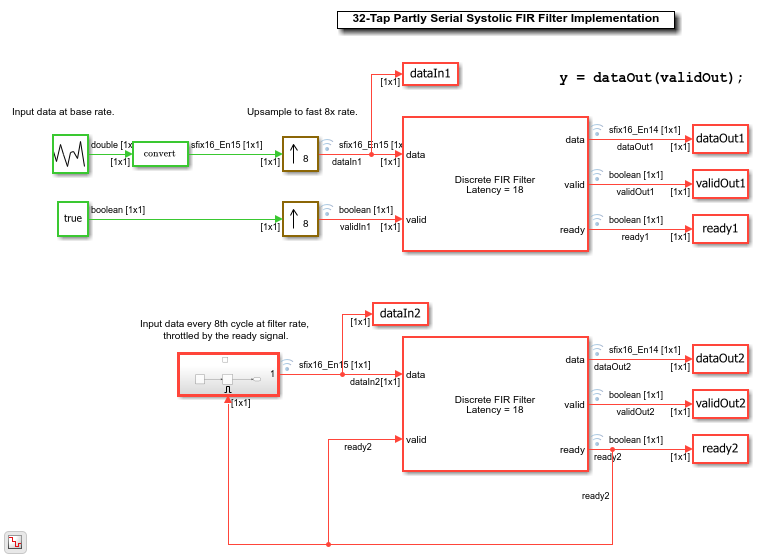### Run Model and Inspect Results

Run the model. Observe the input and output signals in the generated plots. The code to generate the plots is in the `PostSimFcn` callback function.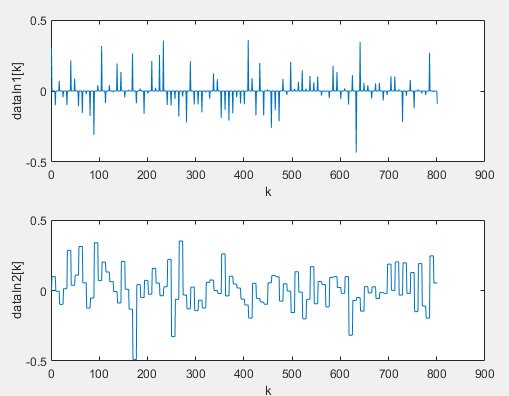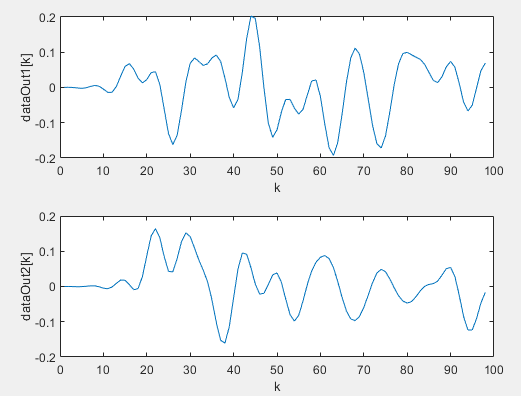Use the model toolbar to open the Logic Analyzer. If the button is not displayed, expand the Review Results app gallery.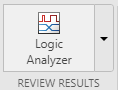Inspect the rising edges of `ready`, `validIn`, and `validOut`.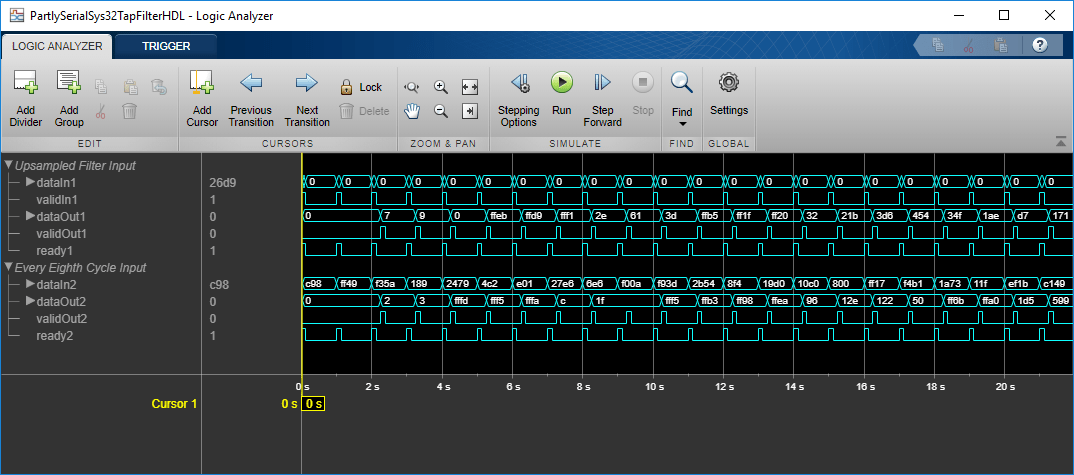### Generate HDL Code

To generate HDL code from either Discrete FIR Filter block, right-click the block and select Create Subsystem from Selection. Then right-click the subsystem and select HDL Code > Generate HDL Code for Subsystem. The two blocks generate the same HDL code.